Science, Maths & Technology

### Become an OU student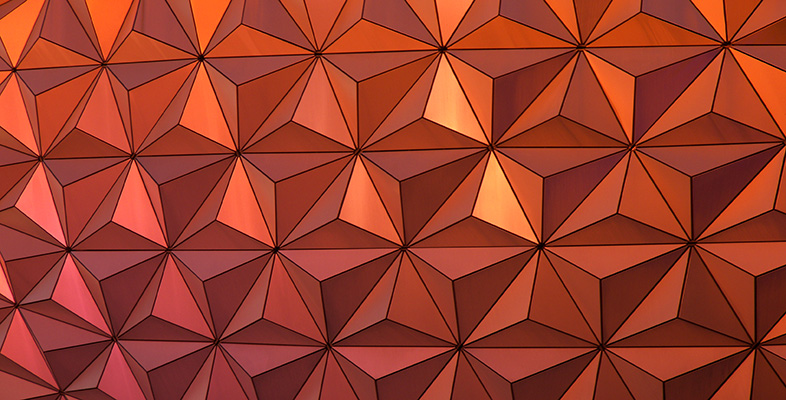Geometry

Start this free course now. Just create an account and sign in. Enrol and complete the course for a free statement of participation or digital badge if available.

# 3.5 Scaling areas and volumes

In OpenLearn course Diagrams, graphs and charts [Tip: hold Ctrl and click a link to open it in a new tab. (Hide tip)] you saw how a scale is used on plans of houses and other structures. The scale makes it possible to take a length on the plan and calculate the corresponding length in reality. The scale can also be used to convert between areas on the plan and real areas. Moreover, if a three-dimensional scale model is made, it is possible to use the scale to convert between volumes in the model and the real volumes.

## Example 16

Dudley's and June's hobby is constructing dolls' houses. They decide to make a model of their own house, using a scale in which 1 cm on the model represents 20 cm on their real house.

They are making the curtains for the model. The window in the real dining room measures 240 cm by 120 cm. What is the area of the real window and the area of the window in the model? How many times greater is the real area?

The real window has an area of 240 cm × 120 cm = 28 800 cm2. It might be easier to think of this in square metres, that is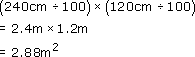To find the dimensions of the window in the model, divide the real lengths by 20 as the scale is 1 cm to 20 cm: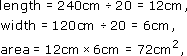or in square metres,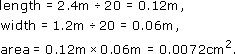Now find the number of times that the area of the real window exceeds the area of the window in the model:

working in square centimetres 28 800 ÷ 72 = 400,

or

working in square metres 2.88 ÷ 0.0072 = 400.

As the real lengths are 20 times greater than those on the model, the areas are 202 (= 400) times greater.

This example has demonstrated a general result:

To scale areas, multiply or divide by the scale squared.

## Example 17

Dudley and June have a cold-water tank in their loft, which has a capacity of 250 litres. If they make a scale model of the tank, what will its capacity be?

Just as areas must be multiplied or divided by the scale squared, so volumes (and capacities) must be multiplied or divided by the cube of the scale. Here the capacity of the real tank must be divided by 203 (= 8000). Therefore

capacity of model tank = 250 litres ÷ 8000 = 0.03125 litres.

As there are 1000 cm3 in one litre,

capacity = 0.03125 × 1000 cm3 = 31.25 cm3.

A check on this value can be made by considering the volume of the real water tank. If it is assumed that the full tank holds exactly 250 litres, the volume of the tank would be at least 250 × 1000 cm3 = 250 000 cm3.

The question does not give the dimensions of the real tank, but to produce this volume, the dimensions might perhaps be 50 cm by 50 cm by 100 cm. (Note that 50 × 50 × 100 = 250 000.) The dimensions of the model of such a tank would be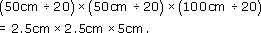So

volume of model tank = 31.25 cm3.

This example illustrates a general result:

To scale volumes, multiply or divide by the scale cubed.